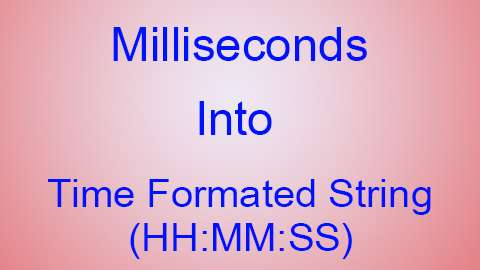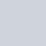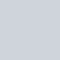# Convert Milliseconds (ms) into Time Formatted String (HH:MM:SS) in Java\AndroidPublished

on

Try this following Method to Convert Milliseconds (ms) into Time Formatted String (HH:MM:SS) in Java\Android.

You just need to Pass the Time in Milliseconds.

``````public String msToTime(long ms)
{
long mm = 0;
long ss = 0;
long hh = 0;

ss = ms/1000;
mm = ss/60;
ss = ss%60;
hh = mm/60;
mm = mm%60;

String ssString = String.valueOf(ss);
String mmString = String.valueOf(mm);
String hhString = String.valueOf(hh);

if (mmString.length() == 1)
{
mmString = "0"+mmString;
}
if (ssString.length() == 1)
{
ssString = "0"+ssString;
}
if (hhString.length() == 1)
{
hhString = "0"+hhString;
}

String time = null;

if (hh!=0)
{
time = hhString+":"+mmString+":"+ssString;
}
else {
time = mmString+":"+ssString;
}

return time;
}``````